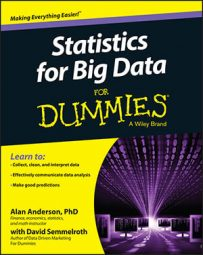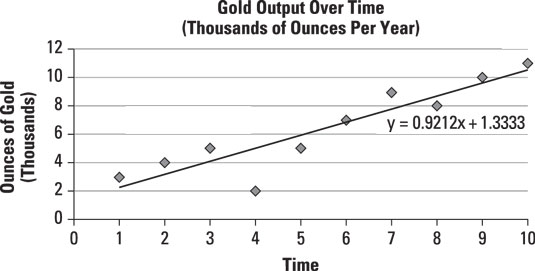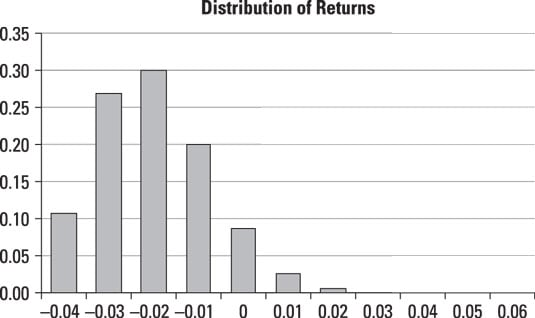##### Statistics for Big Data For DummiesMany different techniques have been designed to forecast the future value of a variable. Two of these are time series regression models and simulation models.

## Time series regression models

A time series regression model is used to estimate the trend followed by a variable over time, using regression techniques. A trend line shows the direction in which a variable is moving as time elapses.

As an example, The figure shows a time series that represents the annual output of a gold mine (measured in thousands of ounces per year) since the mine opened ten years ago.A time series showing gold output per year for the past ten years.

The equation of the trend line is estimated to be

Y = 0.9212X + 1.3333

where

X is the year.
Y is the annual production of gold (measured in thousands of ounces).

This trend line is estimated using regression analysis. The trend line shows that on average, the output of the mine grows by 0.9212 thousand (921.2 ounces) each year.

You could use this trend line to predict the output next year (the 11th year of operation) by substituting 11 for X, as follows:

Y = 0.9212X + 1.3333
Y = 0.9212(11) + 1.3333 = 11.4665

Based on the trend line equation, the mine would be expected to produce 11,466.5 ounces of gold next year.

## Simulation models

You can use simulation models to forecast a time series. Simulation models are extremely flexible but can be extremely time-consuming to implement. Their accuracy also depends on assumptions being made about the time series data's statistical properties.

Two standard approaches to forecasting financial time series with simulation models are historical simulation and Monte Carlo simulation.

### Historical simulation

Historical simulation is a technique used to generate a probability distribution for a variable as it evolves over time, based on its past values. If the properties of the variable being simulated remain stable over time, this technique can be highly accurate. One drawback to this approach is that in order to get an accurate prediction, you need to have a lot of data. It also depends on the assumption that a variable's past behavior will continue into the future.

As an example, this figure shows a histogram that represents the returns to a stock over the past 100 days.A histogram of stock returns.

This histogram shows the probability distribution of returns on the stock based on the past 100 trading days. The graph shows that the most frequent return over the past 100 days was a loss of 2 percent, the second most frequent was a loss of 3 percent, and so on. You can use the information contained within this graph to create a probability distribution for the most likely return on this stock over the coming trading day.

### Monte Carlo simulation

Monte Carlo simulation is a technique in which random numbers are substituted into a statistical model in order to forecast the future values of a variable. This methodology is used in many different disciplines, including finance, economics, and the hard sciences, such as physics. Monte Carlo simulation can work very well but can also be extremely time-consuming to implement. Also, its accuracy depends on the statistical model being used to describe the behavior of the time series.